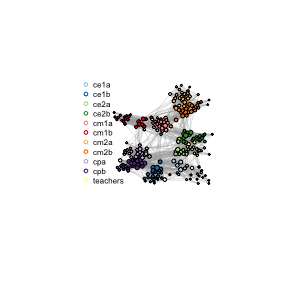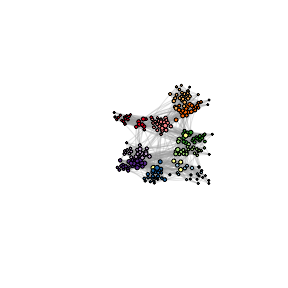# PrimarySchool

## The network of contacts in a primary school

The network of contacts in a primary school was collected an first analyzed in “High-Resolution Measurements of Face-to-Face Contact Patterns in a Primary School” by J. Stehlé et al. in PLOS ONE (2011). This data set records physical interactions between $$226$$ children and $$10$$ teachers from the same primary school over the course of a day. The network data was collected using a system of sensors worn by the participants. This system records the duration of interactions between two individuals facing each other at a maximum distance of one and a half meters.

library(Matrix)
library(gsbm)
library(igraph)
#>
#> Attaching package: 'igraph'
#> The following objects are masked from 'package:stats':
#>
#>     decompose, spectrum
#> The following object is masked from 'package:base':
#>
#>     union
library(RColorBrewer)
library(combinat)
#>
#> Attaching package: 'combinat'
#> The following object is masked from 'package:utils':
#>
#>     combn

data(PrimarySchool)
A <- PrimarySchool$A class <- PrimarySchool$class
n <- dim(A)
class.names <- levels(class)
class.effectifs <- table(class)

The duration of these interactions varies between $$20$$ seconds and two and a half hours. We consider that an physical interaction has actually if the corresponding contact time is greater than one minute. If an interaction of less than one minute is observed, we assume that this observation may be erroneous, and treat the corresponding data as missing. We thus obtain an $$236 \times 236$$ adjacency matrix with $$7054$$ missing entries (including $$236$$ diagonal entries), and $$4980$$ entries equal to $$1$$ (corresponding to $$2490$$ undirected edges). This graph presents strong communities structures, as pupils essentially interact with other pupils of the same class. This phenomenon is exemplified in the following table, which presents the frequency of interaction between individuals according to their respective class.

# Compute frequency of interactions
K = length(class.names)
Q = matrix(rep(0, K*K), ncol = K, nrow = K)
for (k1 in 1:K){
com1 = class.names[k1]
for (k2 in 1:K){
com2 = class.names[k2]
Q[k1, k2] <- mean(A[class == com1, class == com2], na.rm = TRUE)
Q[k2, k1] <- Q[k1, k2]
}
}
Q <- round(Q, 2)
colnames(Q) <- class.names
rownames(Q) <- class.names
print(Q)
#>          ce1a ce1b ce2a ce2b cm1a cm1b cm2a cm2b  cpa  cpb teachers
#> ce1a     0.68 0.07 0.07 0.01 0.00 0.00 0.00 0.00 0.03 0.03     0.10
#> ce1b     0.07 0.83 0.02 0.00 0.01 0.00 0.00 0.00 0.05 0.08     0.11
#> ce2a     0.07 0.02 0.90 0.21 0.00 0.02 0.00 0.01 0.03 0.04     0.10
#> ce2b     0.01 0.00 0.21 0.94 0.05 0.03 0.00 0.03 0.02 0.00     0.13
#> cm1a     0.00 0.01 0.00 0.05 0.97 0.08 0.02 0.07 0.00 0.01     0.09
#> cm1b     0.00 0.00 0.02 0.03 0.08 0.88 0.01 0.03 0.02 0.01     0.05
#> cm2a     0.00 0.00 0.00 0.00 0.02 0.01 0.88 0.29 0.00 0.00     0.08
#> cm2b     0.00 0.00 0.01 0.03 0.07 0.03 0.29 0.93 0.00 0.00     0.08
#> cpa      0.03 0.05 0.03 0.02 0.00 0.02 0.00 0.00 0.97 0.15     0.04
#> cpb      0.03 0.08 0.04 0.00 0.01 0.01 0.00 0.00 0.15 0.97     0.09
#> teachers 0.10 0.11 0.10 0.13 0.09 0.05 0.08 0.08 0.04 0.09     0.29

Then, we visualize the network.

# Vertex labels
v.label <- rep(NA, n)

# Graph without NA's
A.noNA <- matrix(0, ncol = n, nrow = n)
A.noNA[!is.na(A)] <- A[!is.na(A)]
g <- graph_from_adjacency_matrix(A.noNA, mode = "undirected")

# Layout
W<- matrix(0, n, n)
for (i in 1:n){
for (j in 1:n){
if ((!is.na(A[i,j])) && A[i,j]==1){
if (class[i]==class[j] && class[i]!= "teachers"){
W[i,j] <- 2
}
else{
W[i,j] <- 1
}
}
}
}
gw <- graph_from_adjacency_matrix(W, mode = "undirected")
lay <- layout_with_fr(gw)
pal <- brewer.pal(11, "Paired")

# Plot network
plot(g, vertex.label = v.label, vertex.size = (2 + colSums(A, na.rm =T)/5), vertex.color = class, layout = lay, palette = pal, edge.width = 0.3, edge.curved=0.1)

legend("bottomleft", legend = class.names, col = pal, pch = 1, bty = 'n', cex = 0.5, ncol = 1)The MCGD algorithm allows us to detect individuals with abnormal connectivities. When increasing $$\lambda_2$$, or when decreasing $$\lambda_1$$, we decrease the number of nodes that are identified as outliers. However, we note that the set of nodes detected as outliers is stable for parameters values around $$(8.5,8)$$. Moreover, those five nodes are identified as outliers significantly more often than the other nodes when the parameters vary. In the folloing, we therefore use the penalisation $$(\lambda_1, \lambda_2) = (8.5,8)$$.

# Investigate these outliers
T1<-Sys.time()
res <- gsbm_mcgd(A, 8.5, 8, maxit = 100)
T2<-Sys.time()
Tdiff= difftime(T1, T2)
print(paste0("running time : ", Tdiff))
#>  "running time : -1.65716505050659"
outliers <- which(colSums(res$S)>0) no <- length(outliers) outliers.class <- class[outliers] outliers.Q <- matrix(0,nrow = no, ncol = K) for (o in 1:no){ for (k in 1:K){ comk = class.names[k] outliers.Q[o,k] <- mean(A[outliers[o],class == comk], na.rm = T) } print(paste0("node ", outliers[o], " is in class ", outliers.class[o])) print(outliers.Q[o,], 2) print("") } #>  "node 15 is in class ce2b" #>  0.000 0.000 0.556 1.000 0.333 0.091 0.000 0.118 0.000 0.000 0.100 #>  "" #>  "node 30 is in class ce1a" #>  0.929 0.300 0.227 0.077 0.000 0.000 0.048 0.000 0.125 0.150 0.111 #>  "" #>  "node 35 is in class ce1a" #>  0.625 0.238 0.350 0.000 0.059 0.000 0.000 0.050 0.133 0.222 0.111 #>  "" #>  "node 94 is in class ce1b" #>  0.105 0.955 0.056 0.000 0.095 0.000 0.000 0.000 0.222 0.375 0.100 #>  "" #>  "node 180 is in class ce1a" #>  1.00 0.43 0.28 0.00 0.00 0.00 0.00 0.00 0.17 0.12 0.12 #>  "" We investigate the connectivity pattern of these nodes by comparing their connections with different groups to that of other children from their class. We note that children classified as outliers are overall well connected with children from other classes. In a epidemiologic context, they can be considered as super-propagators, who may spread a desease from one group to others. Finally, we show that one can recover the class structure from our estimator $$\widehat{\mathbf{L}}$$. Indeed, it is approximately of rank $$10$$, corresponding to the structure of $$10$$ classes present in the network (the teachers are densely connected with pupils from their class, and scarsely connected with one another, so their community cannot be recovered from the graph). We recover the classes by using a k-means algorithm on the rows of the matrix $$\mathbf{U}$$, where $$\mathbf{U}$$ is a matrix whose columns contain the $$10$$ left singular vectors of $$\widehat{\mathbf{L}}$$. # Compute svd and run k-means L.U <- svd(res$L[-outliers, -outliers], nu = 10)$u est_class <- kmeans(L.U, 10, nstart = 100)$cluster
length(est_class)
#>  231

# Recover the class among the permutations of labels
equ_label = combinat::permn(1:10)
error <- rep(0, length(equ_label))
for (i in 1:length(equ_label)){
error[i] <- sum(class[-outliers] != (class.names[unlist(equ_label[i])])[est_class])
}
error[which.min(error)]
#>  10
class_est <- class.names[unlist(equ_label[which.min(error)])][est_class]
class_est_out <- rep(NA, n)
class_est_out[outliers] <- "outlier"
class_est_out[-outliers] <- class_est
class_est_out <- as.factor(class_est_out)

# Plot network
plot(g, vertex.label = v.label, vertex.size = (2 + colSums(A, na.rm =T)/5), vertex.color = class_est_out, layout = lay, palette = pal, edge.width = 0.3, edge.curved=0.1)class_est_out <- as.factor(class_est_out)

We observe that our method recovers perfectly the class of the children (but for the outliers, who are not classified). Although it cannot classify the teachers as such, we note that it provides a one-to-one mapping between teachers an classes, indicating that it is able to attribute each teacher to her class.